# R语言极值理论：希尔HILL统计量尾部指数参数估计可视化

## 极值理论对样本尾部分布的极值指数的估计方法主要有两类：半参数方法和全 参数方法，前者主要是基于分布尾部的 Hill 估计量，后者则主要基于广义帕累托分布。

， 和

，然后希尔HILL估计为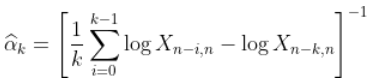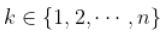。 然后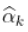在某种意义上满足某种一致性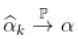,如果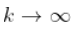，即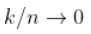（在收敛速度的附加假设下，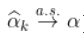）。此外，在附加的技术条件下

`> Q=fuction(p){unro(funion(x) S(x)-(1-p),loer=1,per=1e+9)\$root}`

`> plot(u,Veie(Q)(u),type="l")`

`> X=Vectorize(Q)(runif(n))`

R语言极值理论EVT：基于GPD模型的火灾损失分布分析

hill统计量在这里

`> abline(h=alpha)`

```> for(s in 1:ns){
+ X=Vectorize
+ H=hill
+ hilk=function(k)
+ HilK\[s,\]=Vectorize
+ }```

`> plot(15*(1:10),apply(2,mean)`

# R语言POT超阈值模型和极值理论EVT分析

### 随时关注您喜欢的主题

• 如果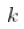太大，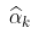是有偏估计量
• 如果太小，是一个不稳定的估计量

（后者来自样本均值的属性：观察越多，均值的波动性越小）。

`> Q=function(p){uniroot(function(x) S(x)-(1-p)}`

`> abline(h=alpha)`

# 但它仅基于一个样本。再次考虑数千个样本，让我们看看 Hill 统计量如何，

Kaizong Ye拓端研究室（TRL）的研究员。

​非常感谢您阅读本文，如需帮助请联系我们！QQ在线咨询

15121130882

0571-63341498

## 关注有关新文章的微信公众号

This will close in 0 seconds